# How to Calculate and Solve for True Stress | The Calculator Encyclopedia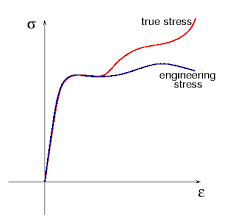The image above represents the true stress.

To compute for the true stress, two essential parameters are needed and these parameters are force (F) and instantaneous area (Ai).

The formula for calculating true stress:

σT = F / Ai

Where;
T = True Stress
F = Force
Ai = Instantaneous Area

Let’s solve an example;
Find the true stress when the instantaneous area is 60 with a force of 25.

This implies that;
F = Force = 25
Ai = Instantaneous Area = 60

σT = F / Ai
σT = 25 / 60
σT = 0.416

Therefore, the true stress is 0.416 Pa.

Calculating the Force when True Stress and Instantaneous Area is Given.

F = Ai x σT

Where;
F = Force
σT = True Stress
Ai = Instantaneous Area

Let’s solve an example;
Find the force when the instantaneous area is 30 with a true stress of 15.

This implies that;
σT = True Stress = 15
Ai = Instantaneous Area = 30

F = Ai x σT
F = 30 x 15
F = 450

Therefore, the force is 450.

Calculating the Instantaneous Area when True Stress and Force is Given.

Ai = F / σT

Where;
Ai = Instantaneous Area
F = Force
σT = True Stress

Let’s solve an example;
Find the instantaneous area when the force is 440 with a true stress of 22.

This implies that;
σT = True Stress = 22
F = Force = 440

Ai = F / σT
Ai = 440 / 22
Ai = 20

Therefore, the instantaneous area is 20.

Nickzom Calculator – The Calculator Encyclopedia is capable of calculating the true stress.

To get the answer and workings of the true stress using the Nickzom Calculator – The Calculator Encyclopedia. First, you need to obtain the app.

You can get this app via any of these means:

To get access to the professional version via web, you need to register and subscribe for NGN 1,500 per annum to have utter access to all functionalities.
You can also try the demo version via https://www.nickzom.org/calculator

Once, you have obtained the calculator encyclopedia app, proceed to the Calculator Map, then click on Material & Metallurgical under Engineering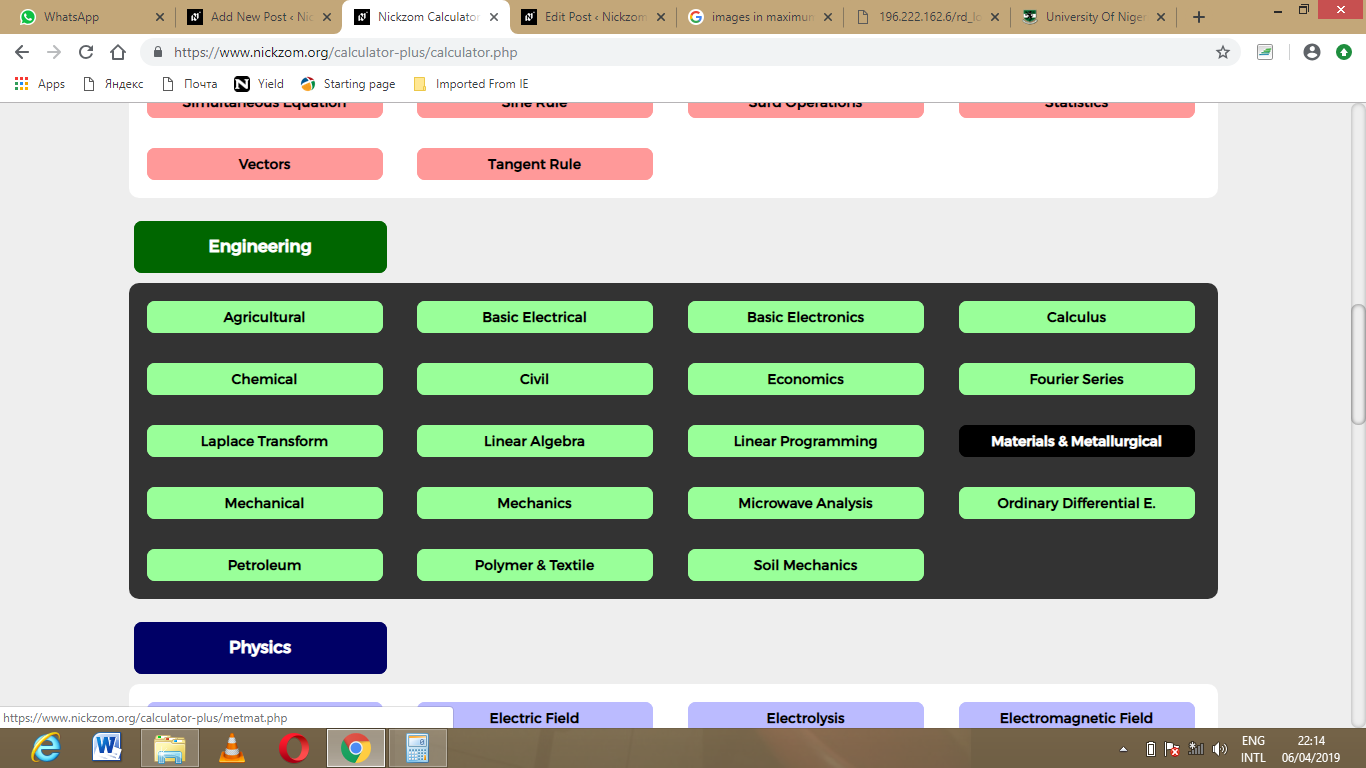Now, Click on Mechanical Properties under Material & Metallurgical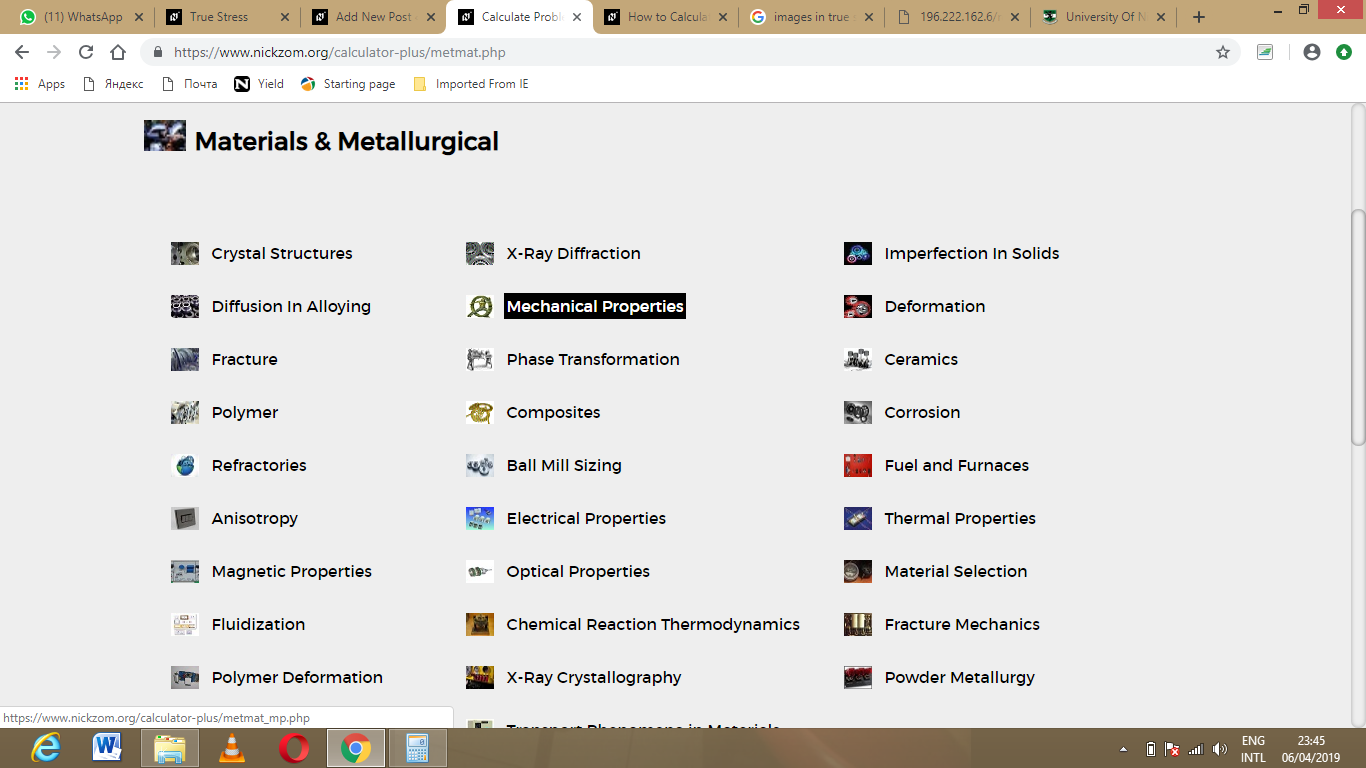Click on True Stress under Material & Metallurgical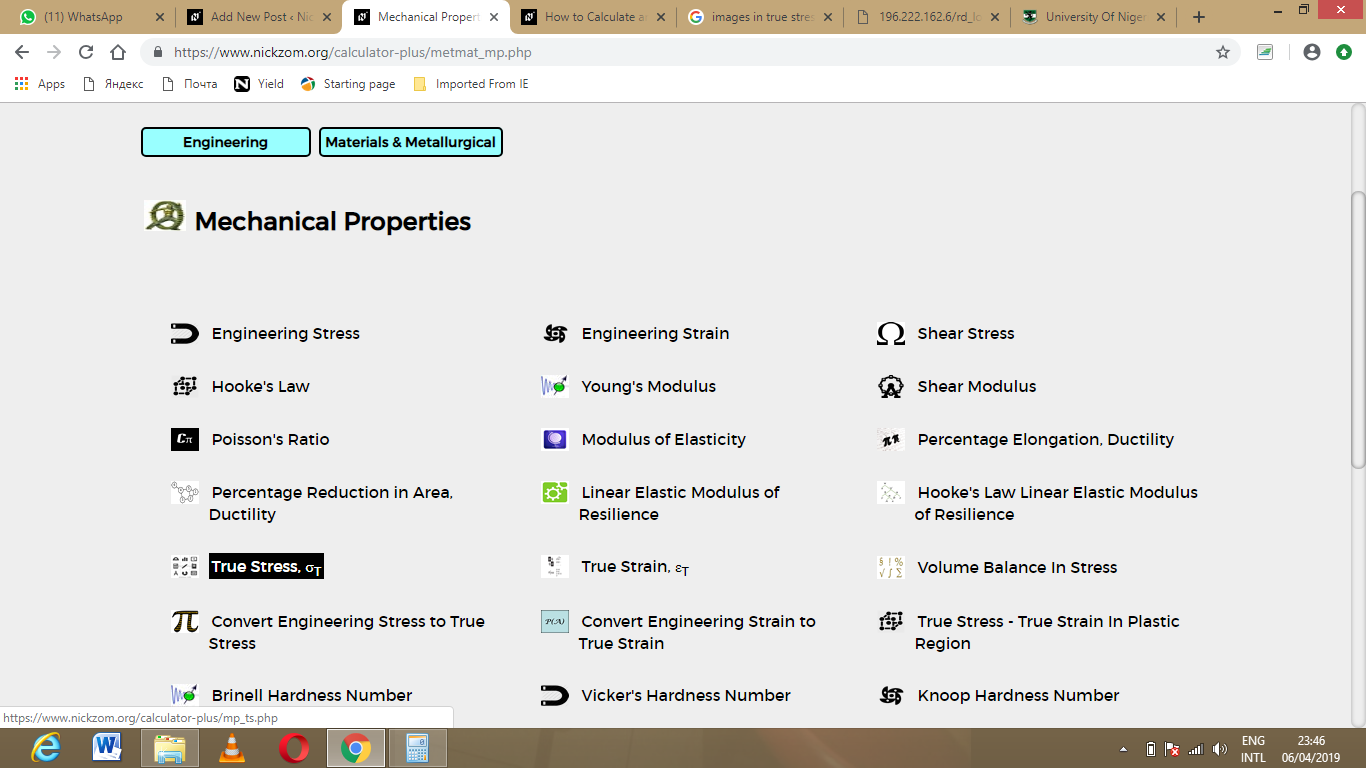The screenshot below displays the page or activity to enter your value, to get the answer for the true stress according to the respective parameter which are the force (F) and instantaneous area (Ai).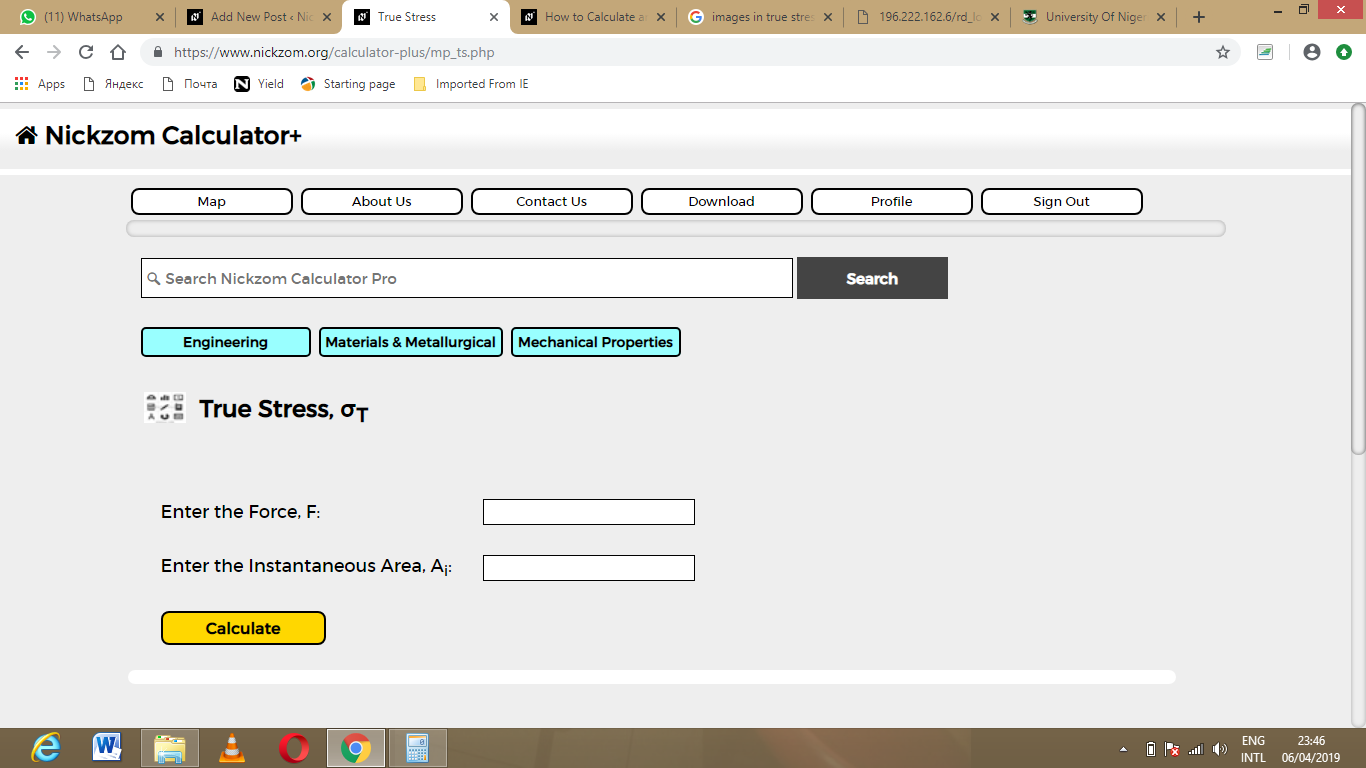Now, enter the value appropriately and accordingly for the parameter as required by the force (F) is 25 and instantaneous area (Aiis 60.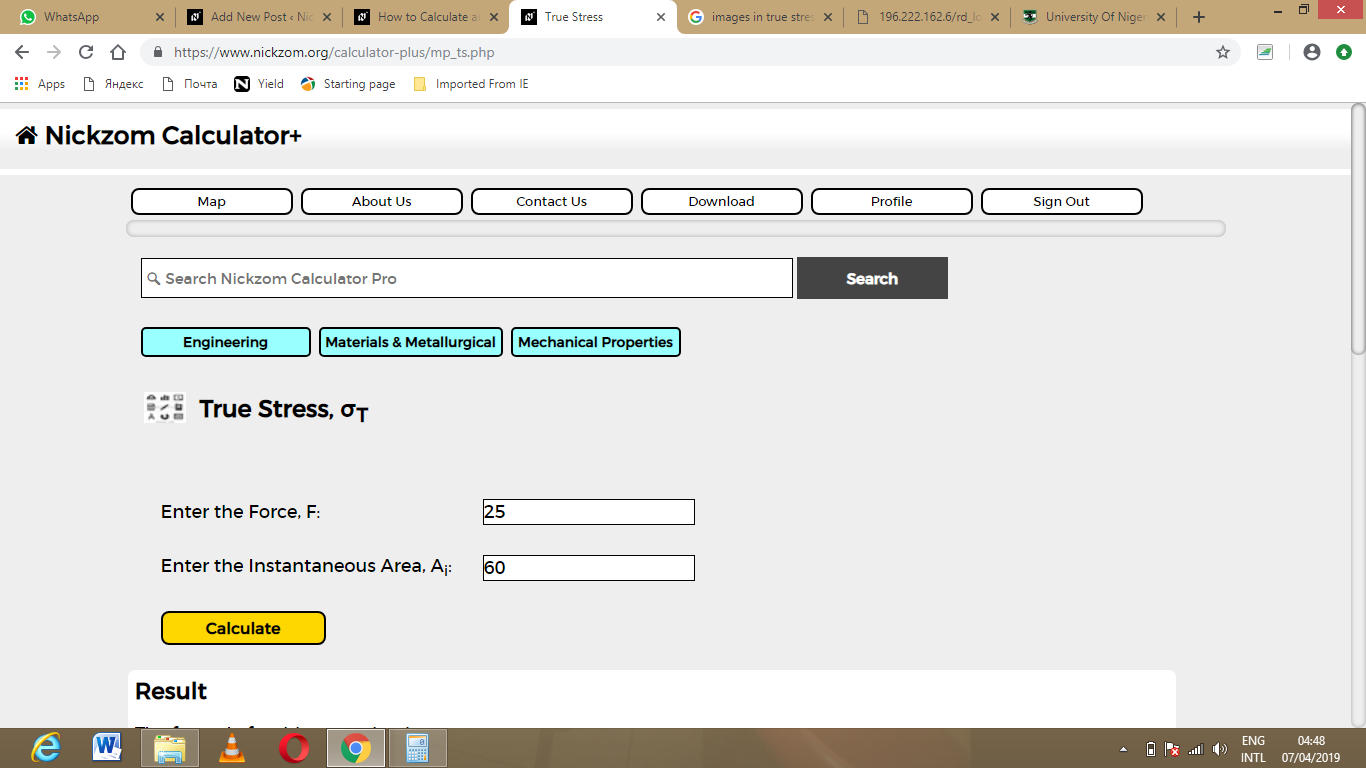Finally, Click on Calculate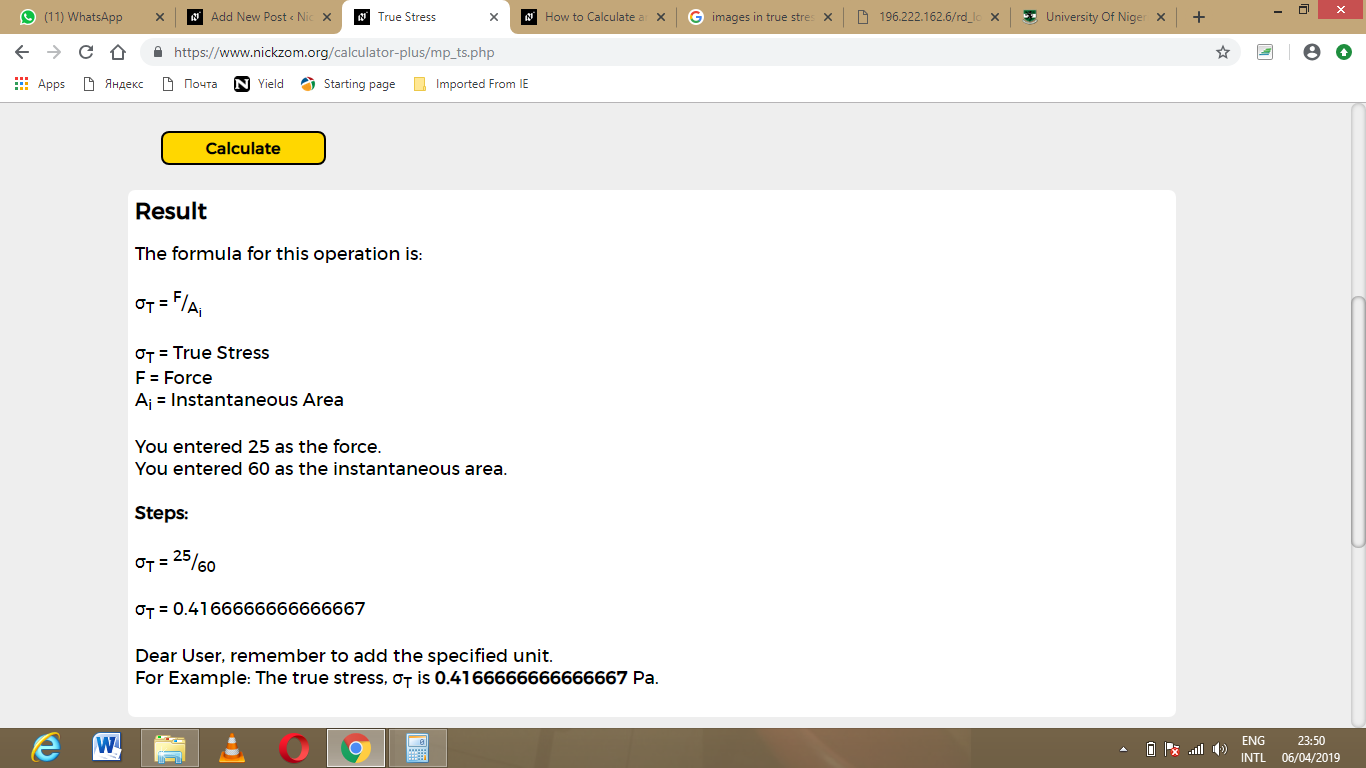As you can see from the screenshot above, Nickzom Calculator – The Calculator Encyclopedia solves for the true stress and presents the formula, workings and steps too.